Pullback of connection on a vector bundle

(Redirected from Pullback connection)

Definition

Suppose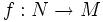$f:N \to M$ is a smooth map between differential manifolds$N$ and$M$. Let$E$ be a vector bundle over$M$, and$f^*E$ denote the pullback of$E$ via$f$ (hence,$f^*E$ is a vector bundle over$N$).

Given a connection$\nabla$ for the vector bundle$E$, we can define a connection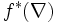$f^*(\nabla)$ for the vector bundle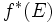$f^*(E)$, called the pullback of$\nabla$, as the unique connection satisfying the following: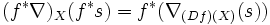$(f^*\nabla)_X(f^*s) = f^*(\nabla_{(Df)(X)}(s))$

This is to be understood as follows. Start with a section$s \in \Gamma(E)$. Take the pullback of$s$ to get a section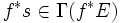$f^*s \in \Gamma(f^*E)$. Then, given a vector field$X$ on$N$,$(f^*\nabla)_X$ should send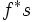$f^*s$ to the pullback via$f$ of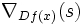$\nabla_{Df(x)}(s)$.

Related facts

Induced connection on submanifold

Further information: induced connection on submanifold

if$M$ is a Riemannian manifold and$N$ is a submanifold, then we can use a linear connection on$M$ to induce a linear connection on$N$. This involves two steps:

• Pull back the connection on$TM$, to the connection on the pullback bundle on$N$ namely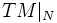$TM|_N$
• Project this to the connection on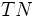$TN$, using the inner product structure on$TM|_N$

Connection along a curve

Further information: connection along a curve

A connection along a curve can be viewed as a special case of a pullback connection, where the pullback is to the interval$(0,1)$.